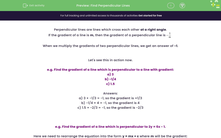# Find Perpendicular Lines

In this worksheet, students will investigate perpendicular lines and find their gradients or equations in the form of numbers or expressions.Key stage:  KS 4

Year:  GCSE

GCSE Subjects:   Maths

GCSE Boards:   Pearson Edexcel, OCR, Eduqas, AQA,

Curriculum topic:   Algebra, Graphs of Equations and Functions

Curriculum subtopic:   Graphs Straight Line Graphs

Difficulty level:#### Worksheet Overview

Perpendicular lines are lines which cross each other at a right angle.

If the gradient of a line is m, then the gradient of a perpendicular line isWhen we multiply the gradients of two perpendicular lines, we get an answer of -1.

Let's see this in action now.

e.g. Find the gradient of a line which is perpendicular to a line with gradient:

a) 3

b) -1/4

c) 1.5

a) 3 × -1/3 = -1, so the gradient is =1/3

b) -1/4 × 4 = -1, so the gradient is 4

c) 1.5 × -2/3 = -1, so the gradient is -2/3

e.g. Find the gradient of a line which is perpendicular to 2y = 6x - 1.

Here we need to rearrange the equation into the form y = mx + c where m will be the gradient:

2y = 6x - 1

y = 3x - 1/2

The gradient of this line is 3.

The gradient of the perpendicular line is -1/3, because 3 × -1/3 = -1.

In this activity, we will investigate perpendicular lines and find their gradients or equations in the form of numbers or expressions.

### What is EdPlace?

We're your National Curriculum aligned online education content provider helping each child succeed in English, maths and science from year 1 to GCSE. With an EdPlace account you’ll be able to track and measure progress, helping each child achieve their best. We build confidence and attainment by personalising each child’s learning at a level that suits them.

Get started